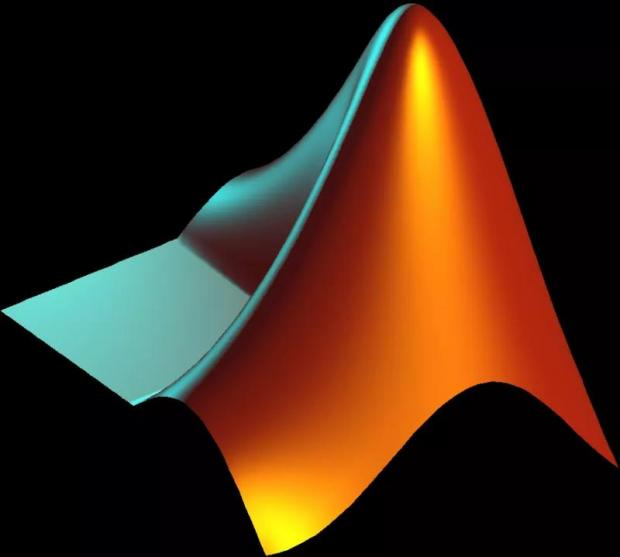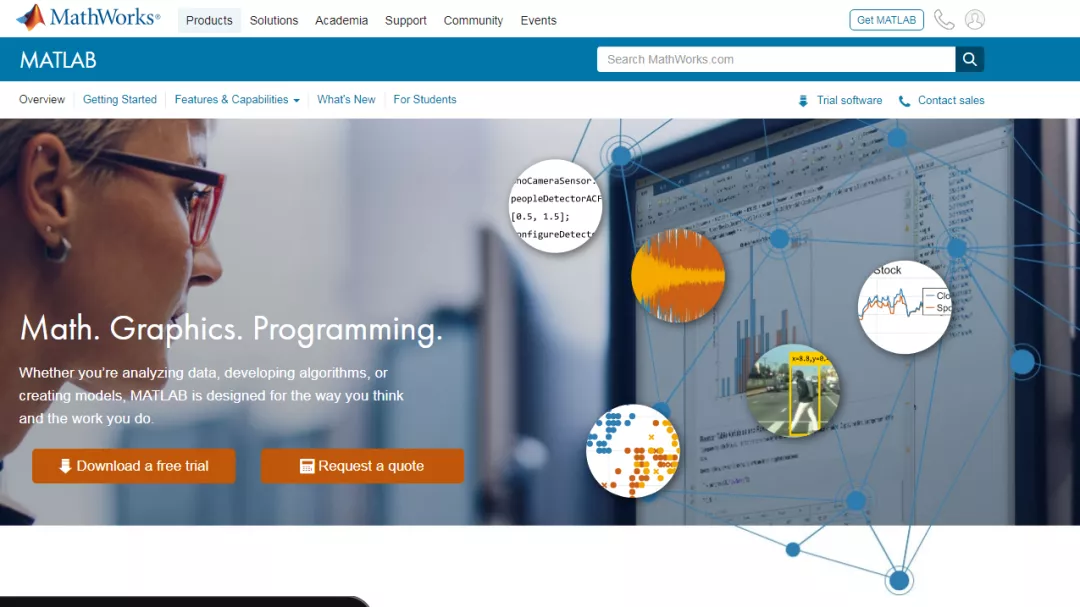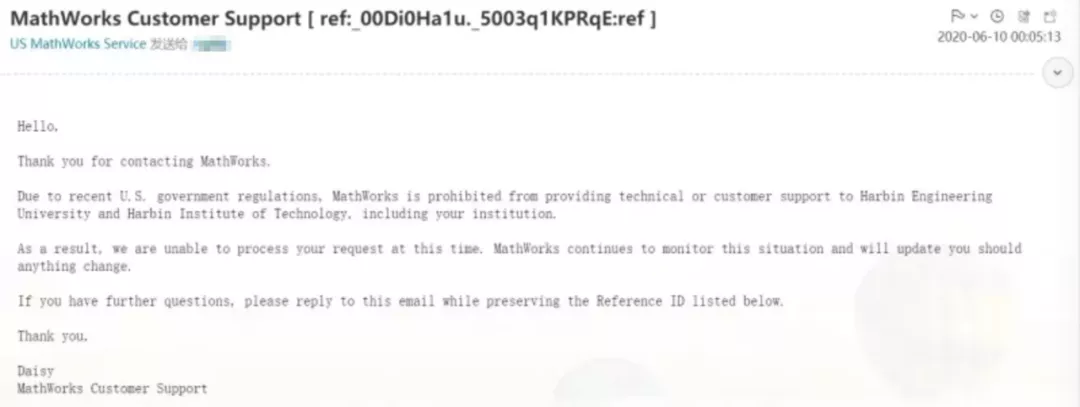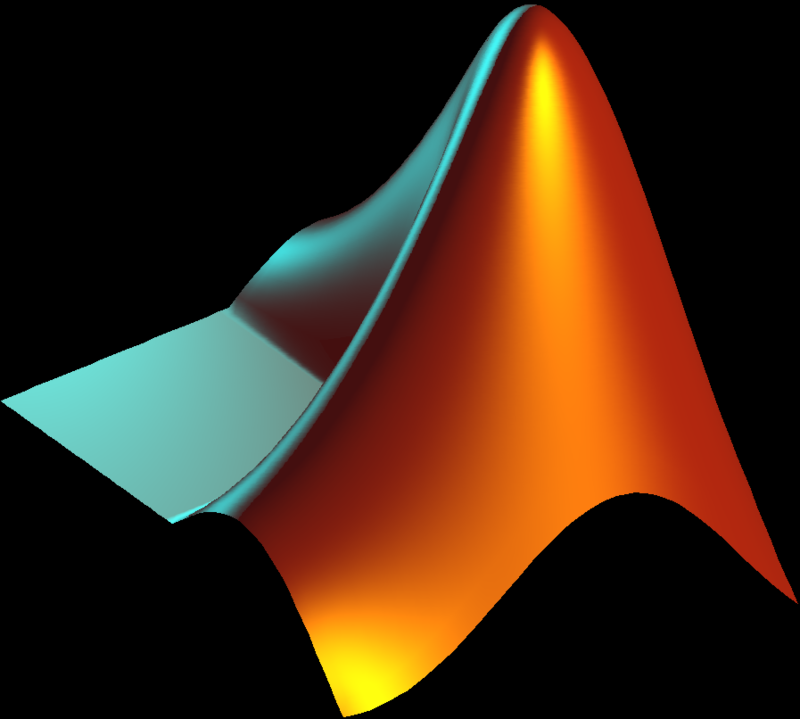MATLAB不能使用，有无可行的替代方案，图片来自cgtn.com

“迫于美国政府的政策，Mathwork s将禁止向哈尔滨工业大学、哈尔滨工程大学提供技术以及客户支持服务。” 6月10日，美国软件公司 Mathworks 客户支持部门的员工在一封给哈工大学生的回复邮件中表示。Mathwork禁止哈工大、哈工程师生使用

MATLAB 软件被禁对工科领域的研究有什么影响？如何应对诸如此类的限禁令…… 《知识分子》向多位理工科的学者以及学生了解他们在教研和学习中对 MATLAB 等工具的使用情况，或许为此事件提供更多探讨的观点与启示。

MATLAB 在国内理工科院校使用情况如何？MATLAB软件标志，图源wikipedia

MATLAB 的全称是矩阵实验室（MATrix LABoratory），它是美国Mathwork s公司旗下的一款商业数学软件，其本身主要用于数值计算，并利用众多附加工具箱（Toolbox）进行功能拓展，涵盖数学和优化、控制系统设计和分析、图像处理等领域。此外，MATLAB 还有功能强大的配套软件包 Simulink，应用于系统模拟等方面。

“受疫情影响，我无法返校，原本的实验部分便改为了仿真，这部分工作主要在 MATLAB 仿真平台中完成。” 哈工大电气学院应届毕业生陶亮（化名）对《知识分子》表示，在MATLAB 仿真平台上，陶亮绘制相关函数的三维图，使用 Simulink 搭建仿真模型等。

“此外学校的部分教学工作，也是涉及MATLAB工具，比如《信号系统》，还有些课程报告需要MATLAB搭建仿真模型。” 陶亮继续补充道， “但学校教学工作涉及的仿真软件还是比较多样化的，也会用到一些专业相关性极强的软件。”

MATLAB 或许只是理工科的众多使用工具之一。当然，选择使用什么工具也跟不同的研究人员的研究方向相关，但总体来看，这类研究工具的使用是多元的。

MATLAB不能使用，有无可行的替代方案？

“近几年，我在学生的推荐下才开始使用 MATLAB，之前主要是用Mathematica（一款与MATLAB齐名、美国Wolfram公司旗下的数学与工程计算商业软件）来做的。”沈阳介绍到，“一般来说，MATLAB能做的符号计算，Mathematica都能做。”

“数值计算领域的经典教材，如《 Matrix Computation 》，往往采用MATLAB 语言编写，我做数值计算的时候也往往用 MATLAB，这样写出的代码更接近书本或论文里的公式，便于对比、查错和后续使用”，韩峰表示，“我也尝试过将工作环节转换到 Python 上并使用 numpy 和scipy （用Python进行数值计算和数据分析时常用的两个库），但感觉不如用 MATLAB 写来得方便，可能是我习惯了后者的缘故。”

MATLAB被禁用，给我们带来的思考

参考资料

https://www.jiqizhixin.com/articles/2020-06-11-7

https://zh.m.wikipedia.org/zh-cn/MATLAB

制版编辑 | 皮皮鱼MATLAB不能使用，有无可行的替代方案，图片来自cgtn.com

●

●

“迫于美国政府的政策，Mathwork s将禁止向哈尔滨工业大学、哈尔滨工程大学提供技术以及客户支持服务。” 6月10日，美国软件公司 Mathworks 客户支持部门的员工在一封给哈工大学生的回复邮件中表示。Mathwork禁止哈工大、哈工程师生使用

MATLAB 软件被禁对工科领域的研究有什么影响？如何应对诸如此类的限禁令…… 《知识分子》向多位理工科的学者以及学生了解他们在教研和学习中对 MATLAB 等工具的使用情况，或许为此事件提供更多探讨的观点与启示。

MATLAB 在国内理工科院校使用情况如何？MATLAB软件标志，图源wikipedia

MATLAB 的全称是矩阵实验室（MATrix LABoratory），它是美国Mathwork s公司旗下的一款商业数学软件，其本身主要用于数值计算，并利用众多附加工具箱（Toolbox）进行功能拓展，涵盖数学和优化、控制系统设计和分析、图像处理等领域。此外，MATLAB 还有功能强大的配套软件包 Simulink，应用于系统模拟等方面。

“受疫情影响，我无法返校，原本的实验部分便改为了仿真，这部分工作主要在 MATLAB 仿真平台中完成。” 哈工大电气学院应届毕业生陶亮（化名）对《知识分子》表示，在MATLAB 仿真平台上，陶亮绘制相关函数的三维图，使用 Simulink 搭建仿真模型等。

“此外学校的部分教学工作，也是涉及MATLAB工具，比如《信号系统》，还有些课程报告需要MATLAB搭建仿真模型。” 陶亮继续补充道， “但学校教学工作涉及的仿真软件还是比较多样化的，也会用到一些专业相关性极强的软件。”

MATLAB 或许只是理工科的众多使用工具之一。当然，选择使用什么工具也跟不同的研究人员的研究方向相关，但总体来看，这类研究工具的使用是多元的。

MATLAB不能使用，有无可行的替代方案？

“近几年，我在学生的推荐下才开始使用 MATLAB，之前主要是用Mathematica（一款与MATLAB齐名、美国Wolfram公司旗下的数学与工程计算商业软件）来做的。”沈阳介绍到，“一般来说，MATLAB能做的符号计算，Mathematica都能做。”

“数值计算领域的经典教材，如《 Matrix Computation 》，往往采用MATLAB 语言编写，我做数值计算的时候也往往用 MATLAB，这样写出的代码更接近书本或论文里的公式，便于对比、查错和后续使用”，韩峰表示，“我也尝试过将工作环节转换到 Python 上并使用 numpy 和scipy （用Python进行数值计算和数据分析时常用的两个库），但感觉不如用 MATLAB 写来得方便，可能是我习惯了后者的缘故。”

MATLAB被禁用，给我们带来的思考参考资料

https://www.jiqizhixin.com/articles/2020-06-11-7

https://zh.m.wikipedia.org/zh-cn/MATLAB

制版编辑 | 皮皮鱼

03361篇文章 1次访问 2小时前更新

• 文章归档
• 最新文章